# 6. (a) Plot four level curves for the surfaces below. Use a meshgrid(-2:0.05:2) i. f(z, y)-9-r2-9y2 for z = 0.5, 1, 2,...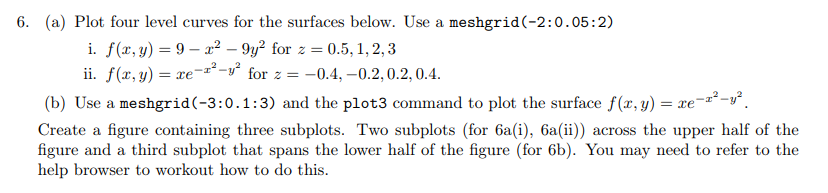6. (a) Plot four level curves for the surfaces below. Use a meshgrid(-2:0.05:2) i. f(z, y)-9-r2-9y2 for z = 0.5, 1, 2, 3 ii. f(x, y) - for0.4,-0.2,0.2,0.4. (b) Use a meshgrid(-3:0.1:3) and the plot3 command to plot the surface f(, y) Create a figure containing three subplots. Two subplots (for 6a(i), 6a(ii)) across the upper half of the figure and a third subplot that spans the lower half of the figure (for 6b). You may need to refer to the help browser to workout how to do this.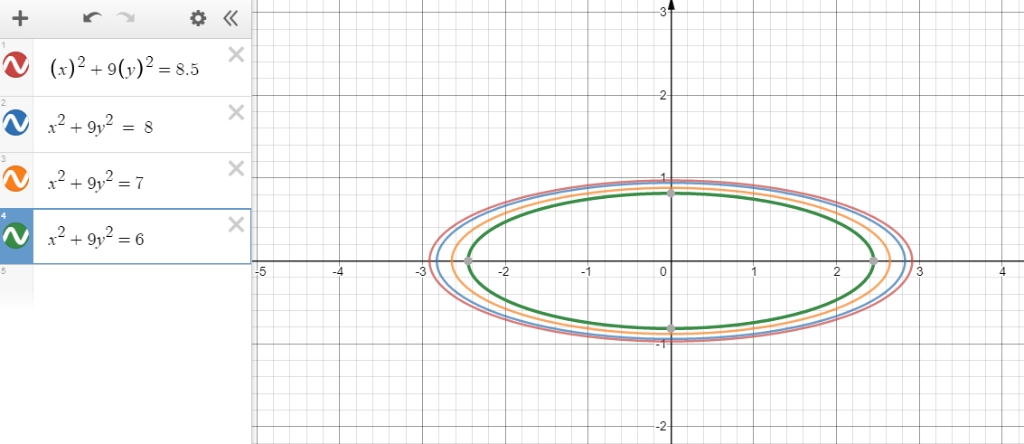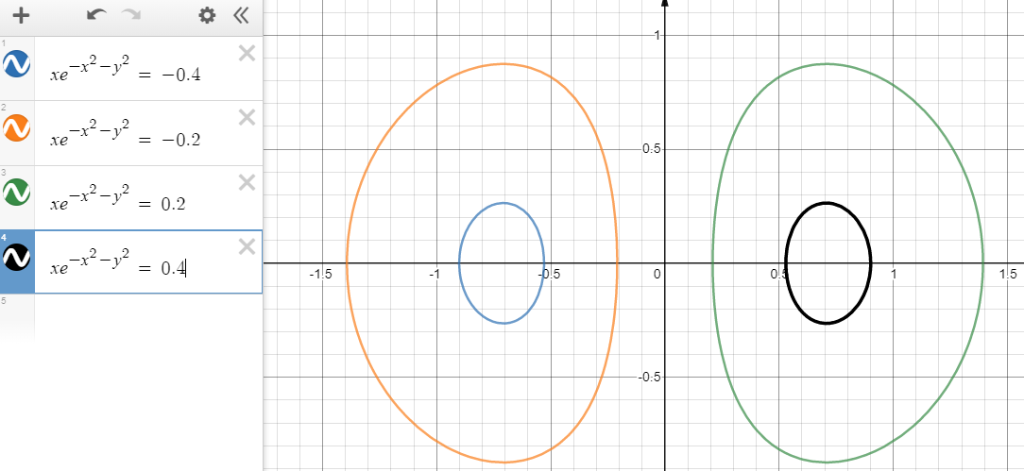b) Plot of the surface.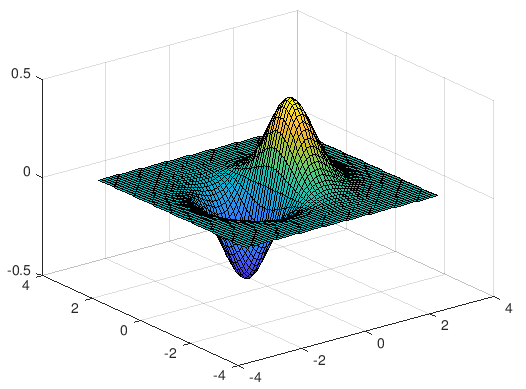##### Add Answer of: 6. (a) Plot four level curves for the surfaces below. Use a meshgrid(-2:0.05:2) i. f(z, y)-9-r2-9y2 for z = 0.5, 1, 2,...
Similar Homework Help Questions
• ### final study guide, please help 1.) Sketch the indicated level curves of the following functions. 0, z = 2, and z = 4 level curves of f(x,y)-хуз. The z =-2, z ). log025 (x + y2 2 level curves of h(...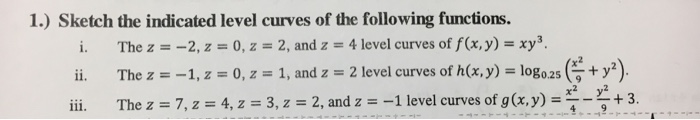final study guide, please help 1.) Sketch the indicated level curves of the following functions. 0, z = 2, and z = 4 level curves of f(x,y)-хуз. The z =-2, z ). log025 (x + y2 2 level curves of h(x,y) -1,2-0, z-, 1, and z ii. The z ii. The z7, z 4,z 3, z 2, and z1 level curves of g(x.y) +3 1.) Sketch the indicated level curves of the following functions. 0, z = 2, and z...

• ### Question 8 (15 marks) Consider the function f: R2 R2 given by 1 (, y)(0,0) f(r,y) (a) Consider the surface z f(x, y). (...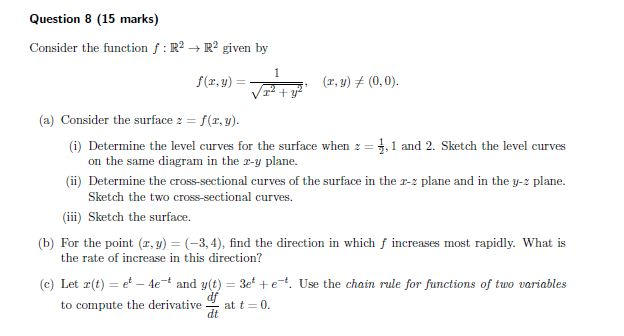Question 8 (15 marks) Consider the function f: R2 R2 given by 1 (, y)(0,0) f(r,y) (a) Consider the surface z f(x, y). (i Determine the level curves for the surface when z on the same diagram in the r-y plane. 1 and 2, Sketch the level curves (i) Determine the cross-sectional curves of the surface in the r-z plane and in the y- plane. Sketch the two cross-sectional curves (iii) Sketch the surface. (b) For the point (r, y)...

• ### question #6 1. Sketch the following surfaces: (a) z-+y2/9 (b) a2 =y2 +22 (c) 2/4+(y-1)2+(z+1)/9 1...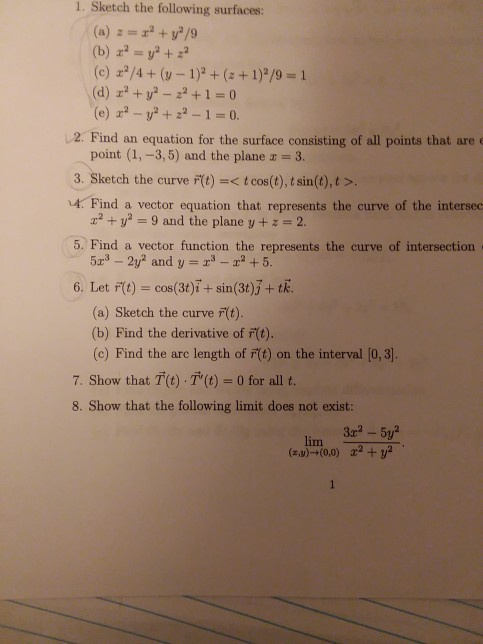question #6 1. Sketch the following surfaces: (a) z-+y2/9 (b) a2 =y2 +22 (c) 2/4+(y-1)2+(z+1)/9 1 (d) r2+y-22+1 0 (e) -y2+-1 0. 2. Find an equation for the surface consisting of all points that are- point (1,-3, 5) and the plane r = 3. 3. Sketch the curve F(t)<t cos(t), t sin (t), t > 4. Find a vector equation that represents the curve of the intersec r2y =9 and the plane y + z = 2. 5. Find a...

• ### 2. Consider the surface -v 9-2r2-r : f(x, y) z (a) What is the domain and range of f? (b) Sketch the level curves f...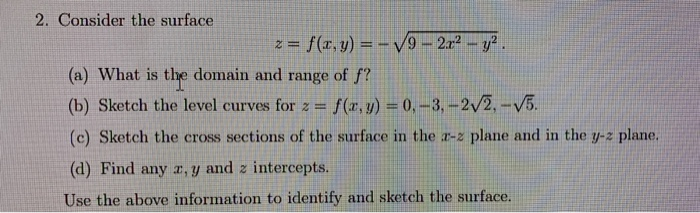2. Consider the surface -v 9-2r2-r : f(x, y) z (a) What is the domain and range of f? (b) Sketch the level curves for 2-f(r,y) -0,-3,-2V2,-v5 (c) Sketch the cross sections of the surface in the r-2 plane and in the y-z plane (d) Find any z, y and z intercepts Use the above information to identify and sketch the surface. 2. Consider the surface -v 9-2r2-r : f(x, y) z (a) What is the domain and range of...

• ### 2. For f(x.y)-9-9x2-y'* a. Sketch the surface z-f(x,y) b. Sketch at least 3 level curves (label each one with its f...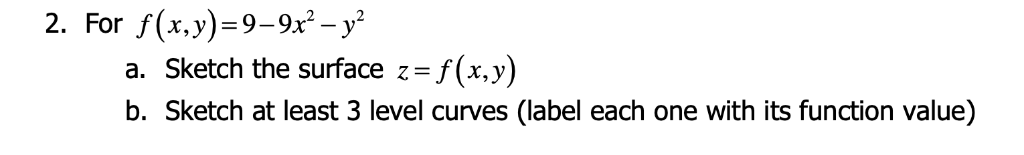2. For f(x.y)-9-9x2-y'* a. Sketch the surface z-f(x,y) b. Sketch at least 3 level curves (label each one with its function value) 2. For f(x.y)-9-9x2-y'* a. Sketch the surface z-f(x,y) b. Sketch at least 3 level curves (label each one with its function value)

• ### 1 point) Match the functions below with their level surfaces at height 3 in the table at the right. 1. f(x,y,z) 22 3x 2.f(x,y,z) 2y +3x 3. f(x, y,z) 2y +3z -2 (You can drag the images to rotate them....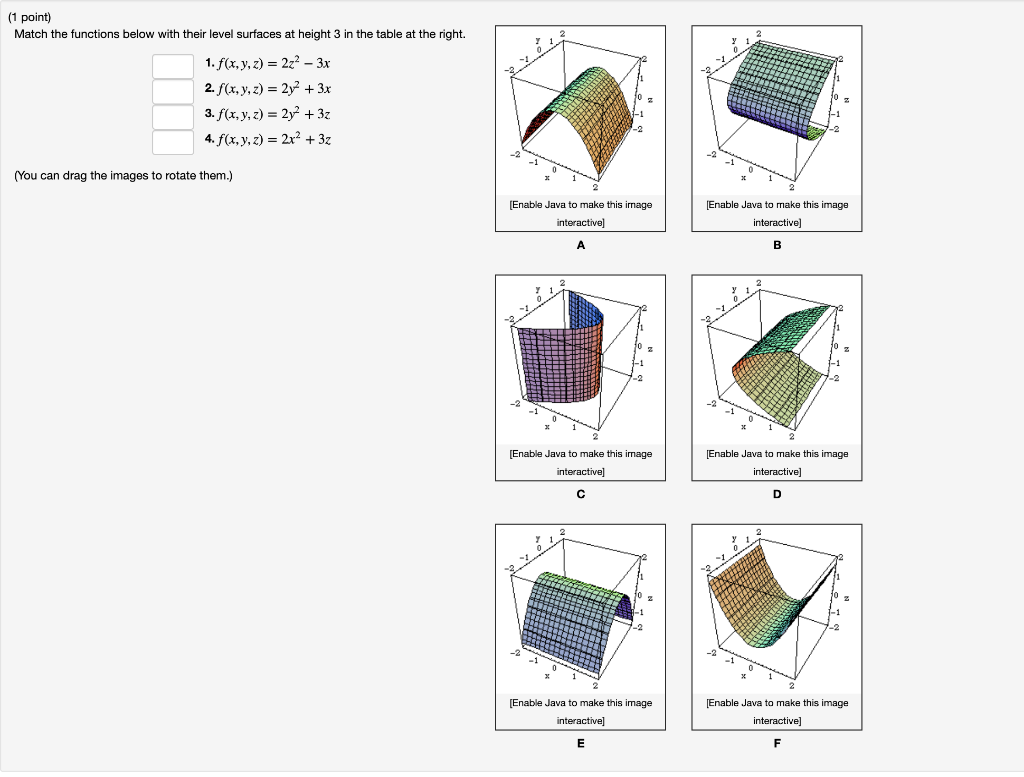1 point) Match the functions below with their level surfaces at height 3 in the table at the right. 1. f(x,y,z) 22 3x 2.f(x,y,z) 2y +3x 3. f(x, y,z) 2y +3z -2 (You can drag the images to rotate them.) Enable Java to make this image Enable Java to make this image interactive] Enable Java to make this image Enable Java to make this image Enable Java to make this image Enable Java to make this image interactive] 1 point)...

• ### 1 Use Stokes' theorem to evaluate the integrals: F(x, y, z) dr a) where F(r, y,z)(3yz,e, 22) and C is the boundary of the triangle i the plane y2 with vertices b) where F(x, y,z (-2,2,5xz) an...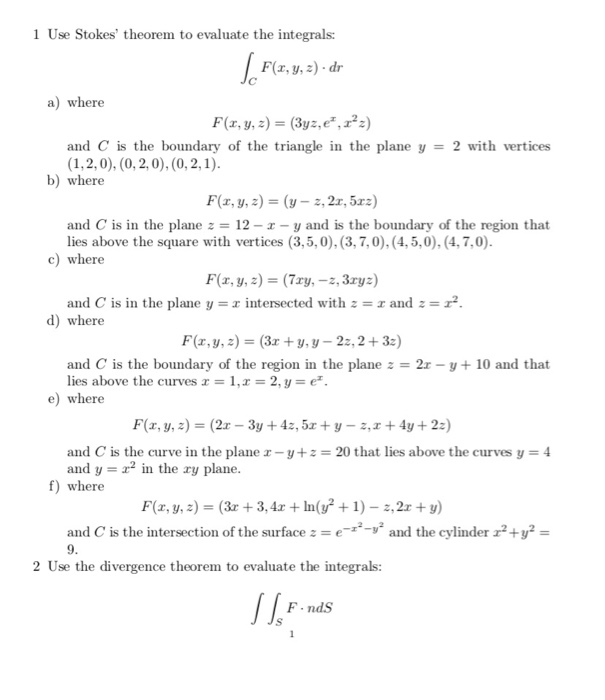1 Use Stokes' theorem to evaluate the integrals: F(x, y, z) dr a) where F(r, y,z)(3yz,e, 22) and C is the boundary of the triangle i the plane y2 with vertices b) where F(x, y,z (-2,2,5xz) and C is in the plane 12- y and is the boundary of the region that lies above the square with vertices (3,5, 0), (3,7,0),(4,5,0), (4,7,0) c) where F(x, y,z(7ry, -z, 3ryz) and C is in the plane y d) where intersected with z...

I'm trying to complete the below problem and I am stuck half way...this is what the problem asks...For this problem, we have four electric charges, q(x,y,z), located in the x-y planeCharge q1(-0.25m, 0m, 0m) = 1 x 10-10 CCharge q2(+0.25m, 0m, 0m) = 1 x 10-10 CCharge q3(0m, -0.25m, 0m) = -1 x 10-10 CCharge q4(0m, +0.25m, 0m) = -1 x 10-10 COver regions parallel to the x-y plane defined for, {-0.2 = x = 0.2} and{-0.2 = y =...

I'm trying to complete the below problem and I am stuck half way...this is what the problem asks...For this problem, we have four electric charges, q(x,y,z), located in the x-y planeCharge q1(-0.25m, 0m, 0m) = 1 x 10-10 CCharge q2(+0.25m, 0m, 0m) = 1 x 10-10 CCharge q3(0m, -0.25m, 0m) = -1 x 10-10 CCharge q4(0m, +0.25m, 0m) = -1 x 10-10 COver regions parallel to the x-y plane defined for, {-0.2 ≤ x ≤ 0.2} and{-0.2 ≤ y ≤...

• ### 1. (25 points) The figure below shows the contour plot of f(x,y)-3 -1 - 2y+y. (Credit for the figure is due to UMich instructors.) 6.00U 6.000 1.5 1.0 0.5 0.0 0.5 1.0 1.5 6.000 2.0-1.5-1.0-0.5 0.0 0....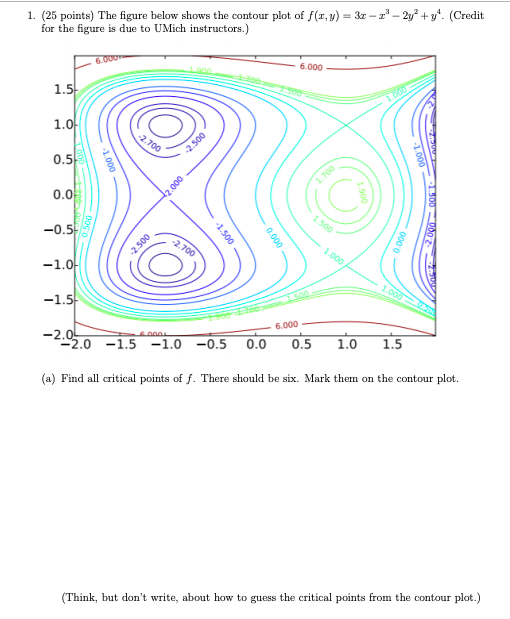1. (25 points) The figure below shows the contour plot of f(x,y)-3 -1 - 2y+y. (Credit for the figure is due to UMich instructors.) 6.00U 6.000 1.5 1.0 0.5 0.0 0.5 1.0 1.5 6.000 2.0-1.5-1.0-0.5 0.0 0.5 1.0 1.5 (a) Find all critical points of f. There should be six. Mark them on the contour plot. (Think, but don't write, about how to guess the critical points from the contour plot.) (b) Find f-,) v(,),and fp()-fy(, y) (c) Try to...

Need Online Homework Help?